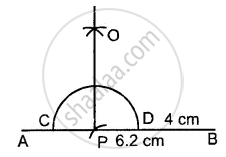# Draw a Line Segment Ab = 6.2 Cm. Mark a Point P in Ab Such that Bp = 4 Cm. Through Point P Draw Perpendicular to Ab. - Mathematics

Sum

Draw a line segment AB = 6.2 cm. Mark a point P in AB such that BP = 4 cm. Through point P draw perpendicular to AB.

#### Solution

Steps of Construction :

1. Draw a line segment AB = 6.2 cm
2. Cut off BP = 4 cm
3. With P as the centre and some radius draw arc meeting AB at the points C, D.
4. With C, D as centres and equal radii [each is more than half of CD] draw two arcs, meeting each other at the point O.
5. Join OP. Then OP is perpendicular for AB.Concept: Drawing the Perpendicular Bisector of a Line Segment
Is there an error in this question or solution?

#### APPEARS IN

Selina Concise Mathematics Class 8 ICSE
Chapter 18 Constructions
Exercise 18 (B) | Q 5 | Page 204